Comparing Fractions
Fractions/Division Relationships
Equivalent Fractions
Improper fractions and mixed numbers
Name that fraction
100

Compare 3/4 and 2/3

3/4 is greater than 2/3

100

What is the division statement for 5/8?

5 divided by 8

100

Write an equivalent fraction for 1/2

2/4, 4/8, 5,10, 6/12

100

Draw a representation for 14/4 and write the mixed number.

3 and 2/4

100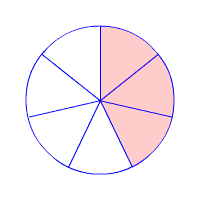Name that fraction

3/7

200

Compare 2/4 and 4/8

they are equal

200

What would the fraction be for 6 divided by 10?

6/10

200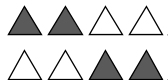Write two fractions that represents this picture

4/8 and 1/2

200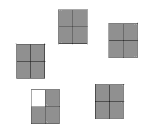What is the mixed number and improper fraction?

4 3/4

19/4

200Name that fraction

5/7

300

Compare 6/8 and 4/5

4/5 is greater than 6/8

300

Write a division statement for 2/4

2 divided by 4

300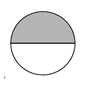Draw a fraction that is equivalent to the representation shown above

300

Write the mixed number for this representation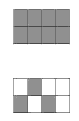1 3/8

300Name the fraction

6/8

400

Compare 3/9 and 1/5

3/9 is greater than 1/5

400

Write the division statement for 14/7

14 divided by 7

400

Draw two representations of 1/4

a picture that represents 1 out of 4 shaded

a picture that shows 3 out of 12 shaded

etc...

400

Write the improper fraction for this representation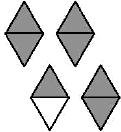7/2

400Name the fraction

4/10

500

Compare 1/2 and 8/9

1/2 is less than 8/9

500

What is the fraction for 15 divided by 5?

15/5

500

Draw an equivalent fraction to represent 1/3

500

Whats the mixed number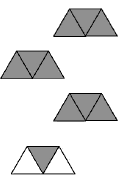3 1/3

500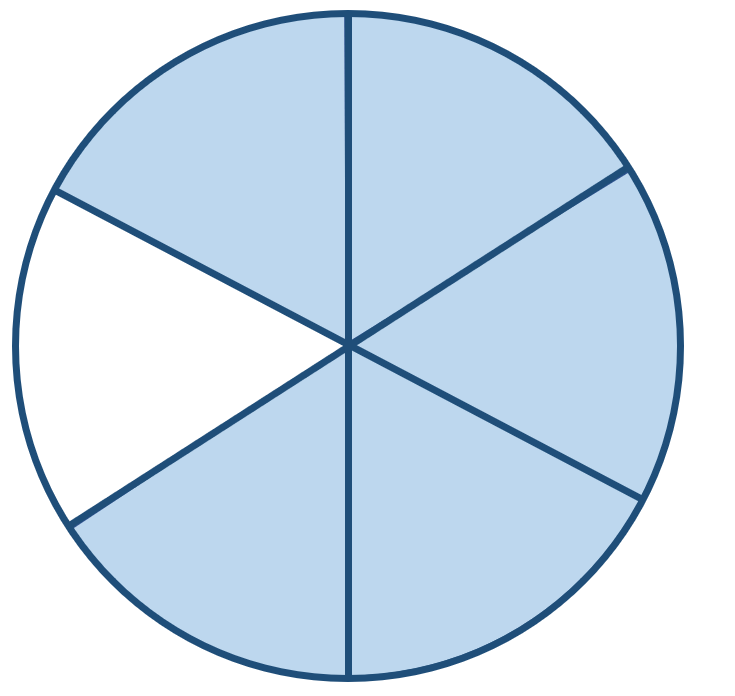Name the fraction

5/6

Click to zoom